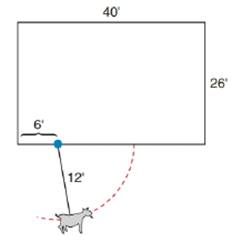Chapter 8.5, Problem 42E### Elementary Geometry for College St...

6th Edition
Daniel C. Alexander + 1 other
ISBN: 9781285195698

#### Solutions

Chapter
Section### Elementary Geometry for College St...

6th Edition
Daniel C. Alexander + 1 other
ISBN: 9781285195698
Textbook Problem
5 views

# A goat is tethered to a barn by a 12 -ft chain. If the chain is connected to the barn at a point 6 ft from one end of the barn, what is the area of the pasture that the goat is able to graze?To determine

To find:

The area of pasture that the goat will be able to graze.

Explanation

Formula:

If r is the radius of circle,

Acircle=πr2.

Calculation:

In the diagram shown below, the shaded area is the pasture that the goat will be able to graze.

The shaded area comprises of a quarter circle of radius 6 ft and a semicircle of radius 12 ft.

Let’s find the area of quarter circle. We know that Acircle=πr2.

Quarter circle is one-fourth part of the entire circle. Hence, the area of quarter circle of radius 6 ft will be 14×πr2.

Let’s substitute r=6 in the above equation to find the area of quarter circle.

Aquarter circle=14×π×62=14×π×36=9π ft2

### Still sussing out bartleby?

Check out a sample textbook solution.

See a sample solution

#### The Solution to Your Study Problems

Bartleby provides explanations to thousands of textbook problems written by our experts, many with advanced degrees!

Get Started

#### In Exercises 14, find the values of x that satisfy the inequality (inequalities). 1. x + 3 2x + 9

Applied Calculus for the Managerial, Life, and Social Sciences: A Brief Approach

#### Find the unit tangent vector for at t = –1.

Study Guide for Stewart's Multivariable Calculus, 8th

#### True or False: The function y = f(x) graphed at the right could be a solution to y = x + y.

Study Guide for Stewart's Single Variable Calculus: Early Transcendentals, 8th